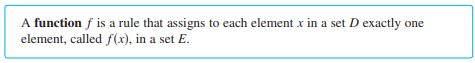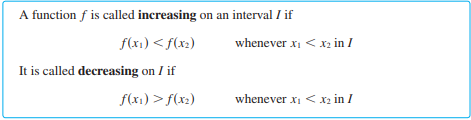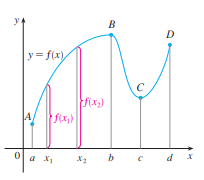Need Help?

Subscribe to Calculus A

###### \${selected_topic_name}
• Notesلو فى functionمعرفة بأكثر من  formulaمختلفة على اجزاء مختلفة من الـ domainالخاص بها ، ، هنسميها piecewise defined function

Four ways to represent a function

● algebraically (by an explicit formula)

● verbally (by a description in words)

● numerically (by a table of values)

● visually (by a graph)

• الدالة التزايدية والدالة التناقصية• اذا كانت  الدالة F(-x) تساوى الدالة F(x)  فان الدالة F دالة زوجية evenواذا كانت  الدالة F(-x)تساوى  - F(x)فان  الدالة fدالة فردية odd